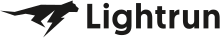• Dev Observability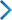• Product• Pricing
• Docs
• Resources• Blog
• Company• Debug Wordle# Stuck on an issue?

Lightrun Answers was designed to reduce the constant googling that comes with debugging 3rd party libraries. It collects links to all the places you might be looking at while hunting down a tough bug.

And, if you’re still stuck at the end, we’re happy to hop on a call to see how we can help out.

## __init__.py files not covered

See original GitHub issue

### Issue Description

Hello !

I just tested codecov on one of my Python project. All my files are correctly present in the file coverage.xml but in the website the init.py files are not present. This lead to false the code coverage. I don’t have a codecov.yml file on my project.

My CI build use codecov 2.0.15

Is it a choice not to include __ init __.py files ?

Here is my coverage report . And the coverage.xml file :

``````<?xml version="1.0" ?>
<coverage branch-rate="0" branches-covered="0" branches-valid="0" complexity="0" line-rate="1" lines-covered="59" lines-valid="59" timestamp="1517859076385" version="4.5">
<!-- Generated by coverage.py: https://coverage.readthedocs.io -->
<!-- Based on https://raw.githubusercontent.com/cobertura/web/master/htdocs/xml/coverage-04.dtd -->
<sources>
</sources>
<packages>
<package branch-rate="0" complexity="0" line-rate="1" name=".">
<classes>
<class branch-rate="0" complexity="0" filename="__init__.py" line-rate="1" name="__init__.py">
<methods/>
<lines>
<line hits="1" number="3"/>
<line hits="1" number="9"/>
<line hits="1" number="12"/>
<line hits="1" number="15"/>
<line hits="1" number="17"/>
<line hits="1" number="20"/>
<line hits="1" number="27"/>
<line hits="1" number="30"/>
<line hits="1" number="33"/>
<line hits="1" number="34"/>
<line hits="1" number="35"/>
<line hits="1" number="37"/>
<line hits="1" number="40"/>
<line hits="1" number="42"/>
<line hits="1" number="45"/>
<line hits="1" number="48"/>
<line hits="1" number="51"/>
<line hits="1" number="52"/>
<line hits="1" number="54"/>
<line hits="1" number="56"/>
<line hits="1" number="58"/>
<line hits="1" number="62"/>
<line hits="1" number="65"/>
<line hits="1" number="71"/>
<line hits="1" number="74"/>
<line hits="1" number="77"/>
</lines>
</class>
<class branch-rate="0" complexity="0" filename="config.py" line-rate="1" name="config.py">
<methods/>
<lines>
<line hits="1" number="2"/>
<line hits="1" number="5"/>
<line hits="1" number="8"/>
<line hits="1" number="9"/>
<line hits="1" number="10"/>
<line hits="1" number="12"/>
<line hits="1" number="13"/>
<line hits="1" number="14"/>
<line hits="1" number="17"/>
<line hits="1" number="20"/>
<line hits="1" number="23"/>
<line hits="1" number="26"/>
<line hits="1" number="27"/>
<line hits="1" number="30"/>
<line hits="1" number="33"/>
<line hits="1" number="36"/>
<line hits="1" number="39"/>
<line hits="1" number="40"/>
<line hits="1" number="43"/>
</lines>
</class>
</classes>
</package>
<package branch-rate="0" complexity="0" line-rate="1" name="resources">
<classes>
<class branch-rate="0" complexity="0" filename="resources/__init__.py" line-rate="1" name="__init__.py">
<methods/>
<lines>
<line hits="1" number="3"/>
<line hits="1" number="4"/>
<line hits="1" number="7"/>
<line hits="1" number="10"/>
<line hits="1" number="12"/>
<line hits="1" number="15"/>
<line hits="1" number="21"/>
</lines>
</class>
<class branch-rate="0" complexity="0" filename="resources/main.py" line-rate="1" name="main.py">
<methods/>
<lines>
<line hits="1" number="3"/>
<line hits="1" number="6"/>
<line hits="1" number="9"/>
<line hits="1" number="10"/>
<line hits="1" number="11"/>
<line hits="1" number="14"/>
<line hits="1" number="16"/>
</lines>
</class>
</classes>
</package>
</packages>
</coverage>
``````

Thanks !

### Issue Analytics

• State: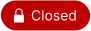• Created 5 years ago
• Reactions:4

####Top GitHub Comments

9reactions
stevepeakcommented, Feb 13, 2018

This question is better suited for `coveragepy` or StackOverflow.

I would check your configuration to make sure they are not ignored in your `.coveragerc` http://coverage.readthedocs.io/en/coverage-4.4.2/config.html

3reactions
spyoungtechcommented, Feb 9, 2018

Ran into this as well. In my case, when I run `coverage`, the `__init__.py` files are included in the coverage report via `coverage report`. But my codecov reports online do not include the `__init__.py` in the source root. `__init__.py` files in submodules are included.

Possibly related to #125 ?

####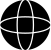Top Results From Across the Web

Coverage.py does not discover tests without init.py file in sub ...
When I run coverage for python, I always need an empty __init__.py file in the tests sub-directory to get coverage to run the...
False positives on empty __init__.py - Sonar Community
While python 3 no longer require the presence of empty __init__.py files to resolve modules imports, the new behavior is not yet supported ......
What is __init__.py file in Python
This article explains why the __init__.py file exists in Python packages. There are two types of packages in python, regular and namespace packages....
Understanding Python imports, __init__.py and pythonpath
Answer: The way we have imported modules in __init__.py above might seem logical to you — after all __init__.py and length.py (or lower.py...
Creating Local Python Packages with __init__.py - codeburst
I'm going to focus on the __init__.py packaging and not namespace ... empty __init__.py file under the print_pkg and time_pkg directories.

####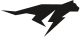Troubleshoot Live Code

Lightrun enables developers to add logs, metrics and snapshots to live code - no restarts or redeploys required.
Start Free

####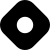Top Related Hashnode Post